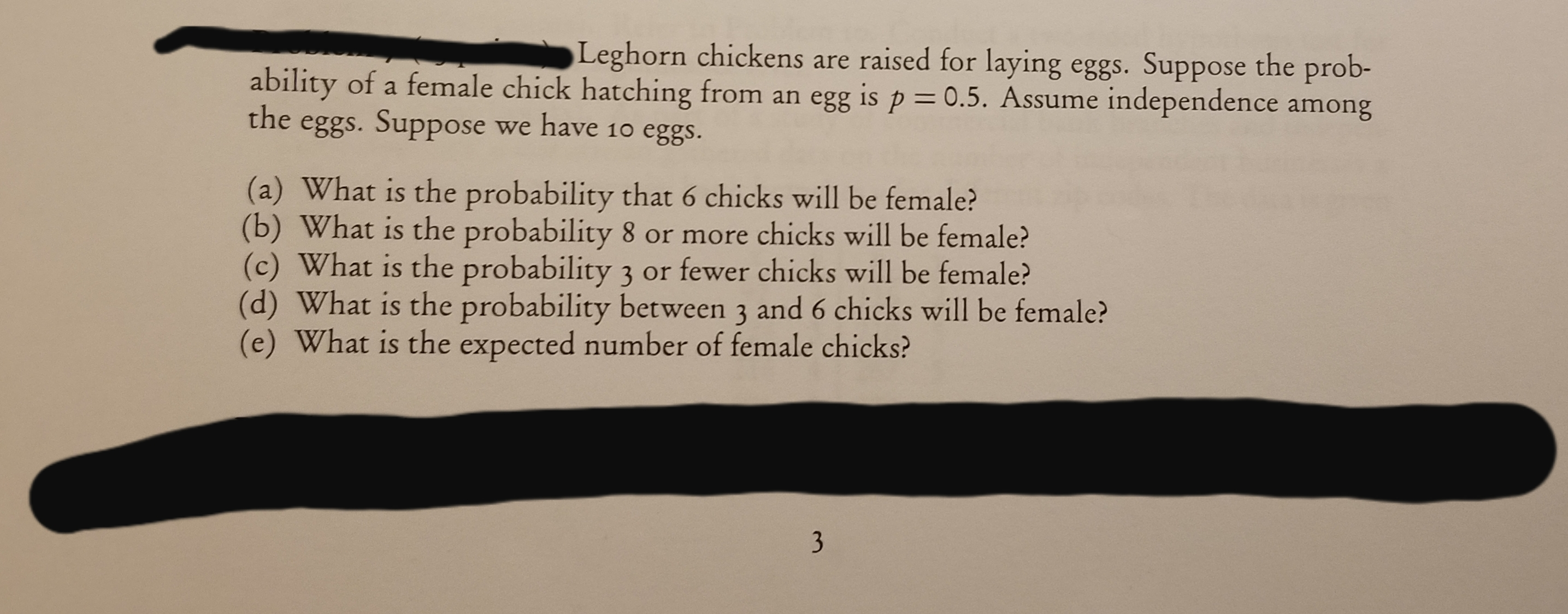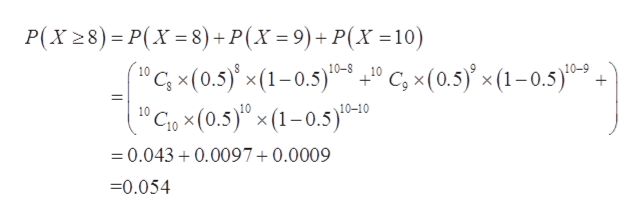# Leghorn chickens are raised for laying eggs. Suppose the prob-ability of a female chick hatching from an egg is p = 0.5. Assume independence amongtheeggs. Suppose we have 10 eggs.(a) What is the probability that 6 chicks will be female?(b) What is the probability 8 or more chicks will be female?(c) What is the probability 3 or fewer chicks will be female?(d) What is the probability between 3 and 6 chicks will be female?(e) What is the expected number of female chicks?3

Question
10 views

Can you do a, b, c?help_outlineImage TranscriptioncloseLeghorn chickens are raised for laying eggs. Suppose the prob- ability of a female chick hatching from an egg is p = 0.5. Assume independence among the eggs. Suppose we have 10 eggs. (a) What is the probability that 6 chicks will be female? (b) What is the probability 8 or more chicks will be female? (c) What is the probability 3 or fewer chicks will be female? (d) What is the probability between 3 and 6 chicks will be female? (e) What is the expected number of female chicks? 3 fullscreen
check_circle

Step 1

The provided information are;

Probability of success (p) = 0.5

Number of trials (n) = 10

Step 2

Consider, X be the random variable that represents the number of chicks that will be female is binomially distributed with the parameters n = 10 and p = 0.5.

(a)

The probability that 6 chicks will be female can be calculated as:

Step 3

(b)

The probability that 8 or more c...help_outlineImage TranscriptioncloseP(X 28) = P(X =8) + P(X = 9)+ P(X =10) %3D %3D "Gx(0.5)* x(1-0.5)*-º C; x(0.5)' x (1–0.5)*- + "Cio x(0.5)" × (1– 0.5)*-10 10-8 +10 10-9 10-10 = 0.043 + 0.0097+0.0009 =0.054 fullscreen

### Want to see the full answer?

See Solution

#### Want to see this answer and more?

Solutions are written by subject experts who are available 24/7. Questions are typically answered within 1 hour.*

See Solution
*Response times may vary by subject and question.
Tagged in